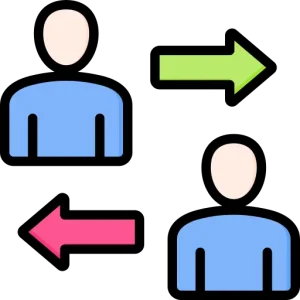# Program to Check whether a given number is Power of 2## Introduction to Check Power of 2

In this article, we will discuss how to write a Python program to check whether a given number is power of 2 or not. Checking if a number is a power of 2 is a common mathematical operation, and Python makes it easy to implement.

The concept is straightforward: a number is considered a power of 2 if it can be expressed as 2^x, where x is a non-negative integer. Recognizing powers of 2 is not only a mathematical curiosity but also a practical necessity in many programming scenarios.

## Understanding the Logic

A number is considered a power of 2 if it can be expressed as 2^x, where x is a non-negative integer. To determine if a number is a power of 2, we can use a simple bitwise operation. Any power of 2 in binary form has only one bit set to 1.

• For example, 2^3 is 8, which is 0b1000 in binary, with only one bit set to 1.

## Examples

Input : n = 64
Output : Yes
Explanation: 26 = 4

Input : n = 32
Output : Yes
Explanation: 25 = 32

## Program to Check whether a given number is Power of 2

Here’s a code snippet :

```# Function to check if a number is a power of 2
def is_power_of_two(num):
# A number is a power of 2 if and only if it has only one bit set in its binary representation.
# Using bitwise operations, we can check if the number has only one '1' bit.
return num > 0 and (num & (num - 1)) == 0

# Get the input number from the user
try:
number = int(input("Enter a number: "))
if is_power_of_two(number):
print(str(number) + " is a power of 2.")
else:
print(str(number) + " is not a power of 2.")
except ValueError:
print("Invalid input. Please enter a valid integer.")
```

### Output :

```Enter a number: 8
8 is a power of 2.
```

### Explanation :

The Python program checks if a given number is a power of 2. It uses bitwise operations to determine if the number has only one ‘1’ bit in its binary representation, which is a characteristic of powers of 2. The program accepts user input, validates it, and then prints whether the number is a power of 2 or not, converting numbers to strings for output without using ‘f’ notation for string formatting.

## How it Works

The core of the logic remains the same as the previous version. The “is_power_of_two” function checks if the number is greater than 0 and whether it has only one ‘1’ bit in its binary representation. We display the results by converting the numbers to strings for concatenation.

##### To wrap it up:

In this tutorial, we’ve explored a Python program to check whether a given number is a power of 2. This program serves as a valuable tool in a programmer’s arsenal, offering an elegant solution to a common mathematical problem. It can be applied in a wide range of scenarios, from optimizing algorithms to enhancing the efficiency of data structures.

### Related Banners

Get PrepInsta Prime & get Access to all 200+ courses offered by PrepInsta in One Subscription

## Get over 200+ course One Subscription

Courses like AI/ML, Cloud Computing, Ethical Hacking, C, C++, Java, Python, DSA (All Languages), Competitive Coding (All Languages), TCS, Infosys, Wipro, Amazon, DBMS, SQL and others